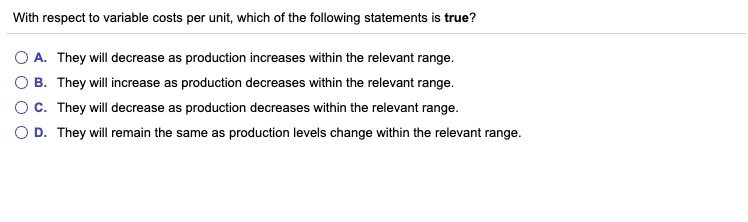# With respect to variable costs per unit. which of the following statements is true?With respect to variable costs per unit, which of the following statements is true? O A. They will decrease as production increases within the relevant range. B. They will increase as production decreases within the relevant range. C. They will decrease as production decreases within the relevant range. D. They will remain the same as production levels change within the relevant range.

Variable cost per unit is always constant in every level of
production, total variable cost increases as production increases.
Total fixed cost is constant, but fixed cost per unit is decreases
as production increases.
Hence, Option “D” is correct. The statement “Variable cost per
unit remains same as production level changes with in the relevant
range” is true.